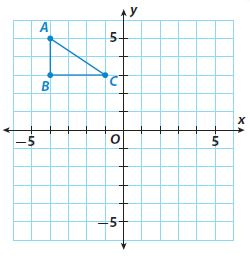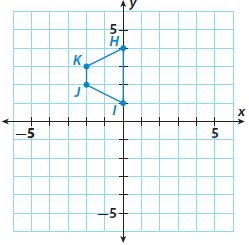Homework Explained - Math Practice 101Dear guest, you are not a registered member. As a guest, you only have read-only access to our books, tests and other practice materials.

As a registered member you can:

Registration is free and doesn't require any type of payment information. Click here to Register.
Go to page:
Chapter 9:Transformations and Congruence; Model Quiz

### 9.1–9.3 Properties of Translations, Reflections, and Rotations

• Question 1

Graph the image of triangle ABC after a translation of 6 units to the right and 4 units down. Label the vertices of the image A’, B’, and C’.• Type below:
• Question 2

On the same coordinate grid, graph the image of triangle ABC after a reflection across the x-axis. Label the vertices of the image A”, B”, and C”.

• Type below:
• Question 3

Graph the image of HIJK after it is rotated 180° about the origin. Label the vertices of the image H’I’J’K’.• Type below:

### 9.4 Algebraic Representations of Transformations

• Question 4

A triangle has vertices at (2, 3), (−2, 2), and (−3, 5). What are the coordinates of the vertices of the image after the translation (x, y) → (x + 4, y − 3)?

• Type below:

### 9.5 Congruent Figures

• Question 5

Vocabulary Translations, reflections, and rotations produce a figure that is _____ to the original figure.

• Type below:
• Question 6

Use the coordinate grid for Exercise 3. Reflect H’I’J’K’ over the y-axis, then rotate it 180° about the origin. Label the new figure H″I″J″K″.

• Type below:

### ESSENTIAL QUESTION

• Question 7

How can you use transformations to solve real-world problems?

• Type below:

Yes, email page to my online tutor. (if you didn't add a tutor yet, you can add one here)